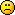# Math Is Fun Forum

Discussion about math, puzzles, games and fun.   Useful symbols: ÷ × ½ √ ∞ ≠ ≤ ≥ ≈ ⇒ ± ∈ Δ θ ∴ ∑ ∫  π  -¹ ² ³ °

You are not logged in.

## #1 2016-04-01 00:31:11

BobRegistered: 2010-06-20
Posts: 9,534

### Regular Icosahedron

The regular icosahedron is one of the five Platonic solids (http://www.mathsisfun.com/geometry/plat … -five.html

It consists of 20 identical equilateral triangles joined 5 at each vertex.  It has 20 faces, 30 edges and 12 vertices. If you put a dot at the centre of each face and join together adjacent face dots the resulting solid is a dodecahedron, having 12 faces, 30 edges and 20 vertices.  These two are 'duals' of each other; a fact I shall make use of later.

If you join opposite vertices, all these lines go through the centre so you can make a sphere that goes through all the vertices.  I shall call the centre to vertex distance the radius of the icosahedron.  In another thread, Monox D. I-Fly asked about this distance, as it is key to working out the volume of the solid.  Here is his diagram:I shall assume a side length of 1, and show how to work out this distance.  Then I'll work out the volume.  I also plan to make a separate thread that shows how to use this to get the volume of a dodecahedron.

Outline of my method.  (1) Obtain expressions for tan(36), cos(36) and sin(36).  I have done that here: http://www.mathisfunforum.com/viewtopic.php?id=22964

(2) Calculate E'C'.  (3) prove that E'C'CA is a rectangle.  (4) Calculate AC' and hence (5) the radius.  (6) Calculate the area of any face.  (7) Calculate the volume of a pyramid with such a triangle as its base and O as its vertex. (8) Hence calculate the volume of the solid.

(2)  Triangle E'D'C' is isosceles so it can be split in two down its line of symmetry, creating a triangle that is 36-54-90.  Thus E'C' is given by

(3)  E'C'CA is a quadrilateral; it has E'C' = AC and E'A = C'C so it is a parallelogram.  But E'C = AC' = 2.radius so it is a parallelogram with equal diagonals, ie. a rectangle.

(4) So, by Pythagoras

(5)

(6) Area of any equilateral triangle:

(7)  I need the perpendicular distance from O to a triangle.

Let M be the midpoint of B'C' and G the centre of triangle PB'C' (third way up median)

(8) every triangle has a pyramid like this and there is no space between them where they meet at O so 20 times the above should give the volume of the icosahedron:

I don't think that is right.So I've got to check to find where I've slipped up.

All errors found and corrected.

Note:

Thus the above simplifies like this:

which is the value given by Monox D. I-Fly here http://www.mathisfunforum.com/viewtopic.php?id=22950

I think I'll rest with that for now.Bob

Children are not defined by school ...........The Fonz
You cannot teach a man anything;  you can only help him find it within himself..........Galileo Galilei
Sometimes I deliberately make mistakes, just to test you!  …………….BobOffline

## #2 2016-04-21 04:55:05

Member
Registered: 2016-04-16
Posts: 1,086

### Re: Regular Icosahedron

Very good calculation.I was not sure about the orientation of various triangles and doubted the analysis but on close scrutiny no hole could be found.Calculation could be shortened by

as OP itself is radius.For curiosity I compared  the volume of icosahedron with volume of sphere.

The difference looked unrealistic at first sight the cut portion having taken away so much volume.The radius of the sphere which accommodated 20 triangles being less than one side of a triangle also looked odd. But looking around the circumference 2 complete sides of triangle like P'B and heights of 4 triangles i.e about only 6 elements along circumference removed the doubt.

Last edited by thickhead (2016-04-22 21:04:09)

{1}Vasudhaiva Kutumakam.{The whole Universe is a family.}
(2)Yatra naaryasthu poojyanthe Ramanthe tatra Devataha
{Gods rejoice at those places where ladies are respected.}

Offline

## #3 2016-04-22 21:06:24

Member
Registered: 2016-04-16
Posts: 1,086

### Re: Regular Icosahedron

It is interesting to calculate some angles.

This is not surprising because when we go along the cicumference say from P to P'
we encounter twice height of triangle and once side of a triangle. I had so much of trouble writing above in LATEX.

I've inserted spaces using space \ space.  Bob Bundy.

Last edited by thickhead (2016-04-23 05:23:16)

{1}Vasudhaiva Kutumakam.{The whole Universe is a family.}
(2)Yatra naaryasthu poojyanthe Ramanthe tatra Devataha
{Gods rejoice at those places where ladies are respected.}

Offline